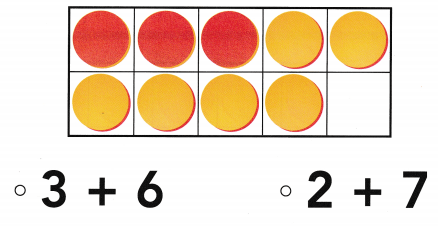# Texas Go Math Kindergarten Lesson 10.3 Answer Key Compose 9

Refer to our Texas Go Math Kindergarten Answer Key Pdf to score good marks in the exams. Test yourself by practicing the problems from Texas Go Math Kindergarten Lesson 10.3 Answer Key Compose 9.

## Texas Go Math Kindergarten Lesson 10.3 Answer Key Compose 9

Essential Question
How do you put together number pairs to make 9?

Explore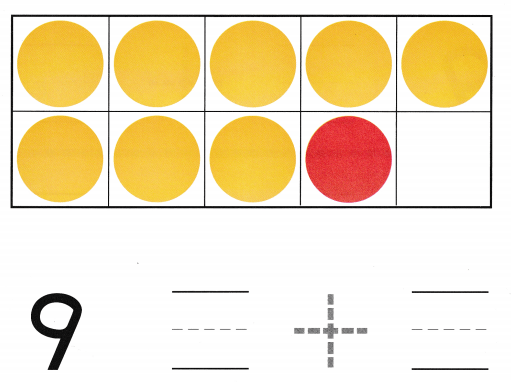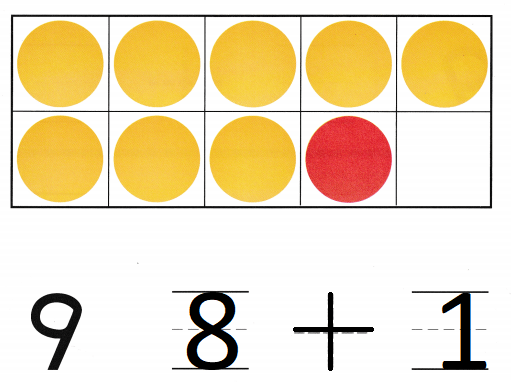Explanation:
Number of yellow counters 8
number of red counters 1
total number of the counters 9
8 + 1 = 9

Directions
Place yellow and red counters in the ten frame as shown. Write the numbers and trace the symbol to show the number pair for 9.

Share and ShowQuestion 1.Explanation:
Number of yellow counters 7
number of red counters 2
total number of the counters 9
7  + 2 = 9

Question 2.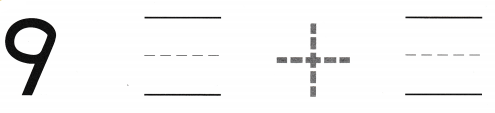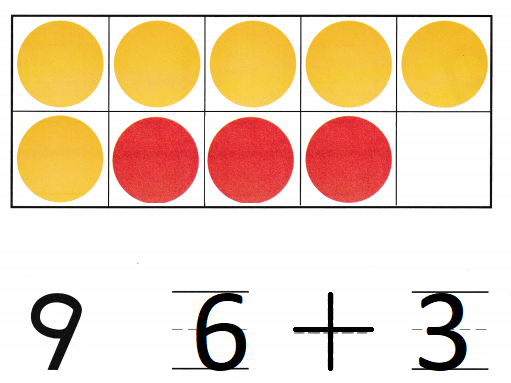Explanation:
Number of yellow counters 6
number of red counters 3
total number of the counters  9
6 + 3 = 9

Question 3.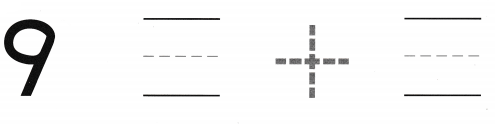Explanation:
Number of yellow counters 5
number of red counters 4
total number of the counters  9
5 + 4 = 9

Directions
Use counters to show number pairs that make 9. 1-3. Write the number pair and trace the symbol. For Exercise 3, color the counters to model the number pair that makes 9.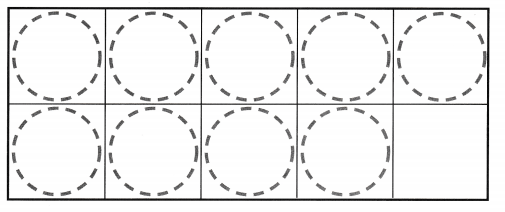Question 4.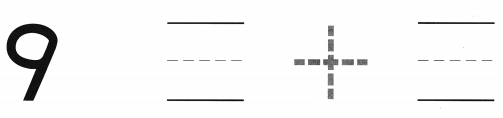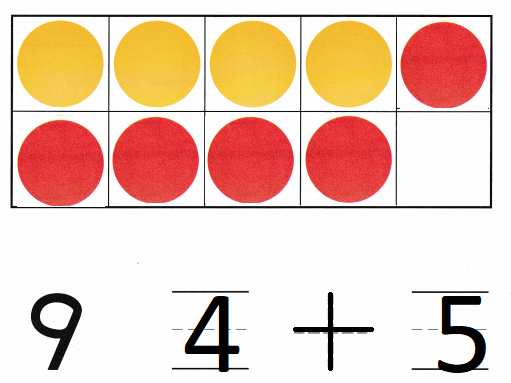Explanation:
Number of yellow counters 4
number of red counters 5
total number of the counters  9
4 + 5 = 9

Question 5.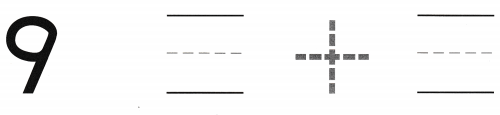Explanation:
Number of yellow counters 3
number of red counters 6
total number of the counters  9
3 + 6 = 9

Question 6.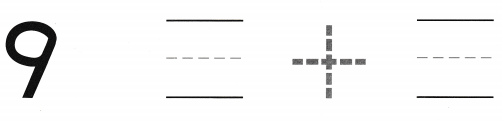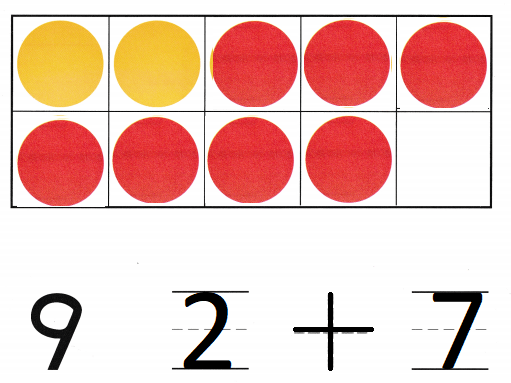Explanation:
Number of yellow counters 2
number of red counters 7
total number of the counters  9
2 + 7 = 9

Directions
Use counters to show number pairs that make 9. 4-6. Write the number pair and trace the symbol. For Exercise 6, color the counters to model the number pair that makes 9.

Home Activity

• Have your child use his or her fingers on two hands to show a number pair for 9.

Problem Solving

Question 7.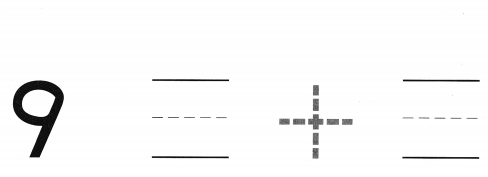Explanation:
Number of yellow counters 1
number of red counters 8
total number of the counters  9
1 + 8 = 9

Question 8.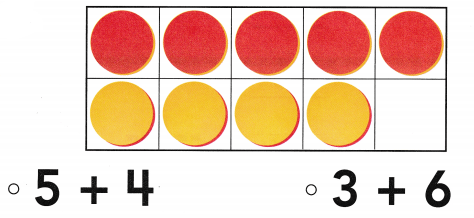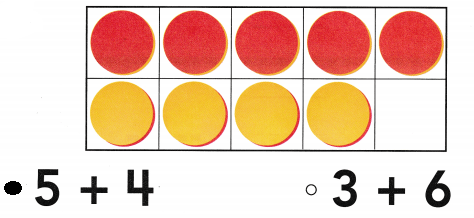Explanation:
Number of yellow counters 4
number of red counters 5
total number of the counters  9
5 + 4 = 9

Directions
7. Shelby has nine counters None of them are red. The rest are yellow. How many are yellow? Draw to solve the problem. Write the numbers and trace the symbol. 8. Choose the correct answer. Count the counters. Which
number pair is shown?

### Texas Go Math Kindergarten Lesson 10.3 Homework and Practice

Question 1.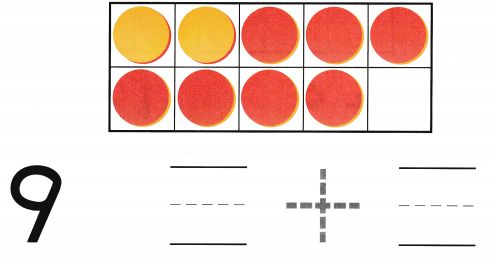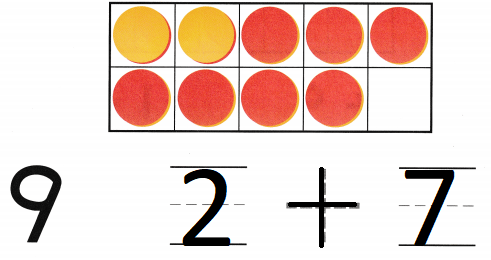Explanation:
Number of yellow counters 2
number of red counters 7
total number of the counters  9
2 + 7 = 9

Question 2.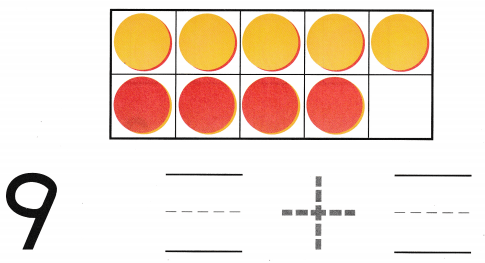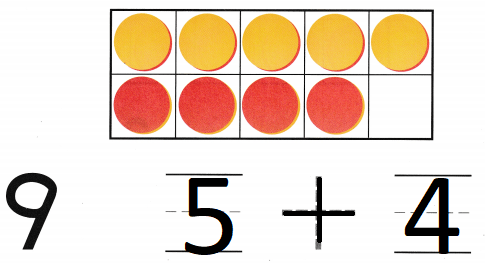Explanation:
Number of yellow counters 5
number of red counters 4
total number of the counters  9
5 + 4 = 9

Directions
1-2. Count the counters in the ten frame that model the number pair that makes 9. Write the number pair and trace the symbol.

Texas Test Prep

Lesson Check

Question 3.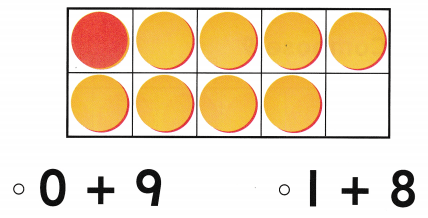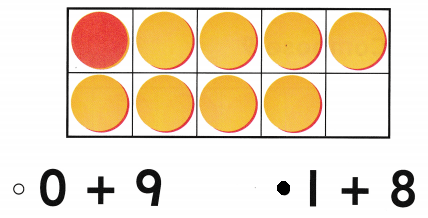Explanation:
Number of yellow counters 8
number of red counters 1
total number of the counters  9
1 + 8 = 9

Question 4.